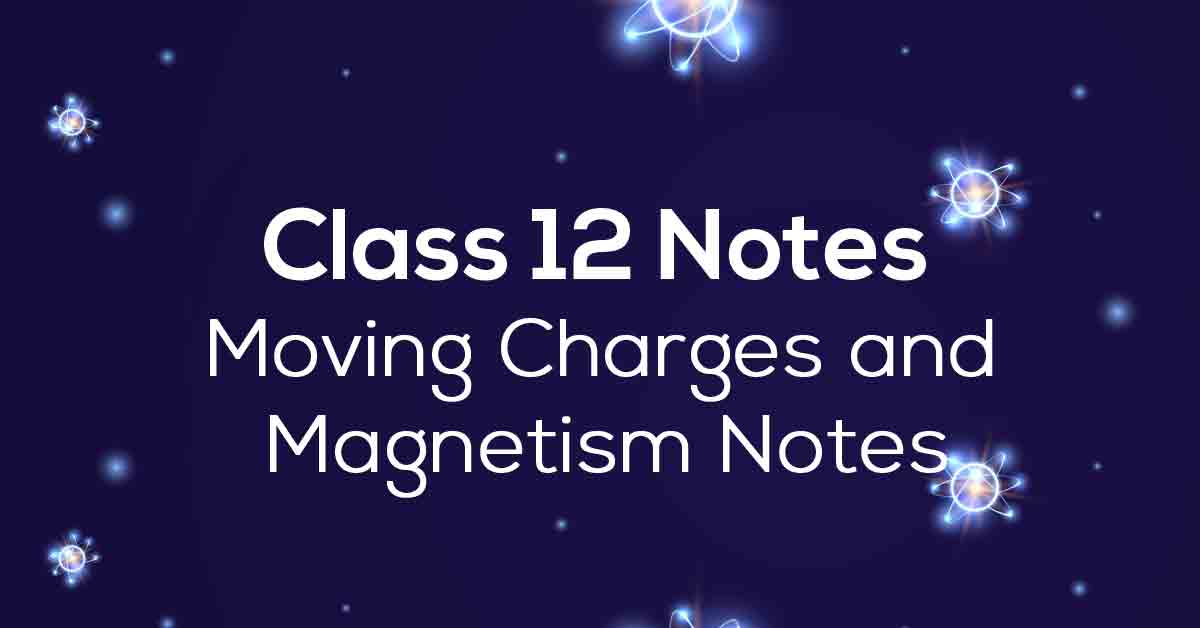Class 12th Physics Moving Charges and Magnetism. NCERT Notes CBSE 2023

# Moving Charges and Magnetism Class 12 Notes# Chapter 4 Moving Charges and Magnetism

The fourth chapter of Physics deals with the chapter Moving Charges and Magnetic Fields. In this chapter, students will learn about magnetic force, motion in magnetic fields, motion in combined electric and magnetic fields, magnetic fields due to an element of Biot Savart's law, and more. These NCERT notes are designed according to the latest class 12 CBSE syllabus.

Physics grade 12 chapter 4 notes NCERT has answers to all questions in the text at the end of the chapter. Physics to help students achieve the results they want. Students taking the board exam should focus on practicing the questions NCERT gives on Vidyakul about moving charge and magnetism. Scroll down to learn more about NCERT notes for Chapter 4 of Grade 12 Physics.

### Points to Remember

Below we have provided some of the important points to remember from NCERT Class 12 Physics chapter 4 to prepare for the board exams:

• Electricity and magnetism have been known to humankind for more than 2000 years.

• In 1820, Danish physicist Hans Christian Oersted discovered the existence of a relationship between electricity and magnetism through a lecture demonstration.

• With further intense experimentation, in 1864, James Maxwell unified and formulated the laws obeyed by electricity and magnetism.

• Through the Moving Charges and Magnetism chapter, students will learn how the magnetic field forces moving charged particles, like electrons, protons, and current-carrying wires and how currents produce magnetic fields.

• Like in the sci-fi movies, students will also learn about a fantastic machine named Cyclotron, created to accelerate charged particles or ions to high energies, and a case study based on that.

• The Moving Charges and Magnetism chapter will have some interesting experiments proving the phenomenon of electromagnetism.

### Topics and Sub-topics

While solving Grade 12 Chapter 4 Physics textbook questions, students may feel the need to review their notes to make sure they are on the right track. However, Vidyakul provides answers to each question in the CBSE Class 12 Chapter 4 Physics textbook based on the latest syllabus.

 Sections Topics Description Ex 4.1 Introduction Ex 4.2 Magnetic Force Ex 4.2.1 Sources and Fields Ex 4.2.2 Magnetic Field, Lorentz Force Ex 4.2.3 Magnetic force on a current-carrying conductor Ex 4.3 Motion in a magnetic field Ex 4.4 Motion in Combined Electric and Magnetic fields Ex 4.4.1 Velocity Selector Ex 4.4.2 Cyclotron Ex 4.5 Magnetic Field due to a current element, Biot-savart law Ex 4.6 Magnetic Field on the Axis Of A Circular Current Loop Ex 4.7 Ampere’s Circuital Law Ex 4.8 The Solenoid and the Toroid Ex 4.8.1 The Solenoid Ex 4.8.2 The Toroid Ex 4.9 Force Between Two Parallel Currents, The Ampere Ex 4.10 Torque on Current Loop, Magnetic Dipole Ex 4.10.1 Torque on a rectangular current loop in a uniform magnetic field Ex 4.10.2 Circular current loop as a magnetic dipole Ex 4.10.3 The magnetic dipole moment of a revolving electron Ex 4.11 The moving coil galvanometer

Download the FREE PDF of Moving Charges and Magnetism Class 12 Notes and start your preparation with Vidyakul!

### Few Important Questions

• An electron is projected into a uniform magnetic field of 3T and moves along a helical path of radius 1 cm with pitch 2π cm. Find the angle of projection of the electron with the magnetic filed.

The angle of projection of the electron with the magnetic filed is 450.

• A cyclotron can accelerate?

Cyclotron, is an apparatus used to accelerate atomic and subatomic particles by an alternating electric field in a constant magnetic field.

• A beam of electron passes, un-deflected through mutually perpendicular electric and magnetic fields. If the electric field is switched off, and the same magnetic field is maintained, the electrons moves in which direction?

The direction of motion of electron must be perpendicular to the both electric and magnetic fields.When electric field is switch off then the magnetic field is perpendicular to the direction of motion of charge particle and therefore, the electrons starts exhibiting circular motion.

### Practice Questions

1. Calculate the magnetic field at the coil’s center if the radius and current flowing in a circular coil of wire having 200 turns are 20 cm and 2 A respectively.?

2. Examine magnitude of the field at a point 60 cm from the wire if the Current flowing in a straight wire is 45 A.?

3. Find out the direction and magnitude at a point 6 m east of the wire in which the current of 70 A is flowing and is passing in the north and south direction.?